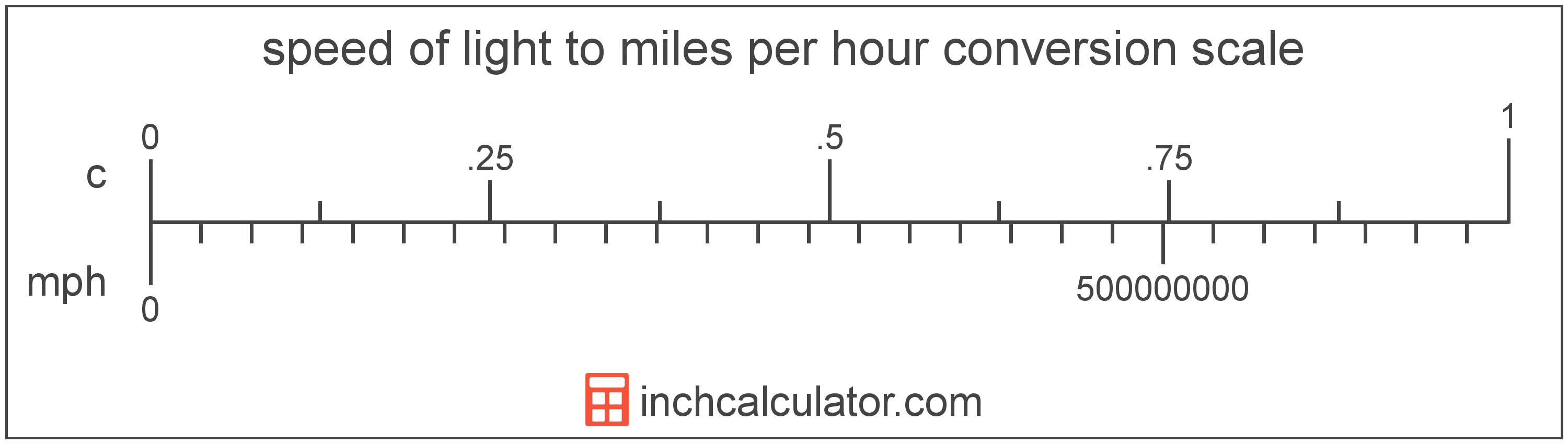# Speed of Light to Miles per Hour Converter

Enter the speed in speed of light below to get the value converted to miles per hour.

Results in Miles per Hour:1 c = 670,616,629.3844 mph

Do you want to convert miles per hour to speed of light?

## How to Convert Speed of Light to Miles per Hour

To convert a measurement in speed of light to a measurement in miles per hour, multiply the speed by the following conversion ratio: 670,616,629.3844 miles per hour/speed of light.

Since one speed of light is equal to 670,616,629.3844 miles per hour, you can use this simple formula to convert:

miles per hour = speed of light × 670,616,629.3844

The speed in miles per hour is equal to the speed in speed of light multiplied by 670,616,629.3844.

For example, here's how to convert 5 speed of light to miles per hour using the formula above.
miles per hour = (5 c × 670,616,629.3844) = 3,353,083,146.922 mphSpeed of light and miles per hour are both units used to measure speed. Keep reading to learn more about each unit of measure.

## What Is the Speed of Light?

The speed of light is equal to exactly 299,792,458 meters per second, or 670,616,629 miles per hour. The definition of the speed of light is actually derived from the most recent 1983 international definition of the meter.

Speed of light can be abbreviated as c; for example, 1 speed of light can be written as 1 c.

## What Are Miles per Hour?

Miles per hour are a measurement of speed expressing the distance traveled in miles in one hour.

The mile per hour is a US customary and imperial unit of speed. Miles per hour can be abbreviated as mph, and are also sometimes abbreviated as mi/h or MPH. For example, 1 mile per hour can be written as 1 mph, 1 mi/h, or 1 MPH.

Miles per hour can be expressed using the formula:
vmph = dmi / thr

The velocity in miles per hour is equal to the distance in miles divided by time in hours.

## Speed of Light to Mile per Hour Conversion Table

Table showing various speed of light measurements converted to miles per hour.
Speed Of Light Miles Per Hour
0.00000001 c 6.7062 mph
0.00000002 c 13.41 mph
0.00000003 c 20.12 mph
0.00000004 c 26.82 mph
0.00000005 c 33.53 mph
0.00000006 c 40.24 mph
0.00000007 c 46.94 mph
0.00000008 c 53.65 mph
0.00000009 c 60.36 mph
0.000000001 c 0.670617 mph
0.00000001 c 6.7062 mph
0.0000001 c 67.06 mph
0.000001 c 670.62 mph
0.00001 c 6,706 mph
0.0001 c 67,062 mph
0.001 c 670,617 mph
0.01 c 6,706,166 mph
0.1 c 67,061,663 mph
1 c 670,616,629 mph

## References

1. National Institute of Standards and Technology, SI Redefinition: Meter, https://www.nist.gov/si-redefinition/meter
2. Wikipedia, Miles per hour, https://en.wikipedia.org/wiki/Miles_per_hour